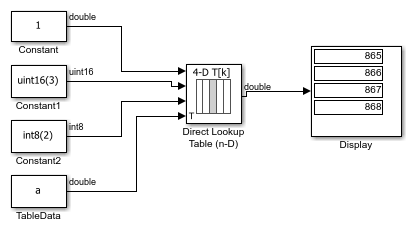Providing Table Data as an Input to the Direct Lookup Table Block

This example shows how to provide table data as an input to the Direct Lookup Table block. In the following model, a is a 4-D array of linearly increasing values that you define with the following model preload function:

a = reshape(1:2800, [4 5 20 7]);

When you run the model, you get the following results:The block labeled TableData feeds a 4-D array to the Direct Lookup Table (n-D) block, with a data type of double. Because the Direct Lookup Table (n-D) block uses zero-based indexing, the output is:

a(:,2,4,3)

The output has the same data type as the table data input signal: double.